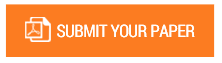RP-27: Formulation of Solutions of a Class of Congruence of Prime-Power Modulus of Higher Degree.

Authors : B. M. Roy.

Volume/Issue : Volume 3 - 2018, Issue 4 - April

Scribd : https://goo.gl/J72Psi

Thomson Reuters ResearcherID : https://goo.gl/3bkzwv

In this paper, a formula to find the solutions of a congruence of prime power modulus of higher degree the type ??≡ ? (??? ??), where p is an odd positive prime integer and n≥ ?,is any positive integer, is established. The formula is of great merit and of time-saving in calculation.The method found in the Mathematicsliterature is time-consuming. It is also found that such a congruence is solvable if ??≡ ? (??? ??) and each congruence has exactly p solutions and in total, there are (p-1) such congruence.

Keywords : Binomial Expansion; Fermat’s Theorem Primepower modulus.

#### CALL FOR PAPERS

Paper Submission Last Date
31 - May - 2022